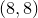# 11.40 move

```move <item number> to <vector> [ rotate <angle> ]
```

The move command allows vector graphics objects to be moved around on the current multiplot canvas. All vector graphics objects placed on multiplot canvases receive unique identification numbers which count sequentially from one, and which may be listed using the list command. The item to be moved should be specified using its identification number. The example

```move 23 to 8,8
```

would move multiplot item 23 to positioncentimeters. If this item were a plot, the end result would be the same as if the command set origin 8,8 had been executed before it had originally been plotted.